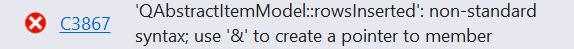# Qtableview enter in column0

• I just need to enter 1,2,3-- in all the rows of column0 means row 0 so 1 like that.

``````    QAbstractItemModel* table1 = ui.tableView->model();
int iRows = table1->rowCount();
int iCols = table1->columnCount();
for (int row = 0;row < iRows;row++) {
int col = 0;
for (int i = 1;i <= iRows;i++) {
QModelIndex index = model->index(row, col);
model->setData(index, i);
}
}
``````

• any other way to do or what changes in the code ?

• ``````QAbstractItemModel* table1 = ui.tableView->model();
for (int i = 0, maxI=table1->rowCount();i <= maxI;++i)
table1->setData(table1->index(i,0), i+1);
``````

• Thanks its working
But when i am adding new row then in that row number is not coming in column 0. ?what other change required

• Smells like you are trying to reinvent the headers... in any case:

``````QObject::connect(table1,QAbstractItemModel::rowsInserted,table1,[table1](const QModelIndex &parent, int first, int last){
for(int i=first;i<=last;++i)
table1->setData(table1->index(i,0,parent), i+1);
});``````

•getting error
i am adding row like this
void tool::on_pushButton_Clicked()
{
model->insertRow(model->rowCount(QModelIndex()));
}

• getting error

Yes it was a typo but the error tells you clearly what to do, you could figure it out...

``````QObject::connect(table1,&QAbstractItemModel::rowsInserted,table1,[table1](const QModelIndex &parent, int first, int last){
for(int i=first;i<=last;++i)
table1->setData(table1->index(i,0,parent), i+1);
});
``````

• @VRonin is it possible to add alpabets in column 0 in every row ?
code--
QAbstractItemModel* table1 = ui.tableView->model();
for (char i = 'A';i <= 'Z';++i)
table->setData(table->index(i, 0), i );

• The second argument of `setData` is what determines what will appear in the cell. You don't need to change the loop that is iterating over the rows:

``````QAbstractItemModel* table1 = ui.tableView->model();
for (int i = 0, maxI=table1->rowCount();i <= maxI;++i)
table1->setData(table1->index(i,0), QChar('A'+i));
``````

• Ok so using setdata
in col 6 i need to perform operation i.e. col6=col5/col4
connect(ui.tableView->model(), SIGNAL(dataChanged(QModelIndex, QModelIndex)),
SLOT(UpdateData(QModelIndex, QModelIndex)));
}
void tool::UpdateData(const QModelIndex& indexA, const QModelIndex& indexB)
{
QAbstractItemModel* table1 = ui.tableView->model();
for (int i = 0, maxI = table1->rowCount();i <= maxI;++i) {
int valor1 =table1->data(table1->index(i, 4)).toInt();
int valor2 = table1->data(table1->index(i, 5)).toInt();
table1->setData(table1->index(i, 6), valor2 / valor1);
}
}
as initially there is no data in col so it throws exception error (/0) how to prevent this
getting exception error

• as initially there is no data in col so it throws exception error (/0)

You already identified the problem, you just need an `if` to work around it

• @VRonin yes i tried using if but not able to solve the problem

• i tried using if

What did you try?

• @VRonin
QAbstractItemModel* table1 = ui.tableView->model();
for (int i = 0, maxI = table1->rowCount();i <= maxI;++i) {
int valor1 =table1->data(table1->index(i, 4)).toInt();
int valor2 = table1->data(table1->index(i, 5)).toInt();
if (valor1==0 || valor2== 0)
valor1 = 16, valor2 = 37;
table1->setData(table1->index(i, 6), valor2 / valor1);
}
i assign some initial values but now every row will update first with some data is there any other way to overcome from exception and this calculation will work?

• You are SOOOOO close to the solution!
2 more hints:

• whatever `valor2` is, it's never a problem, only the denominator causes the div0 error
• you don't need to assign default values, you can just set it to be blank if it would cause an error

• You are SOOOOO close to the solution!
2 more hints:

• whatever `valor2` is, it's never a problem, only the denominator causes the div0 error
yes right
• you don't need to assign default values, you can just set it to be blank if it would cause an error
``````int valor2 = table1->data(table1->index(i, 5)).toInt();
if (valor1==0 || valor2== 0)
table1->setData(table1->index(i, 6), " "); //this is correct way
table1->setData(table1->index(i, 6), valor2 / valor1);
``````

blank in setdata
can you plz tell how to set blank ?
pass `QVariant()` as the second argument to `setData`